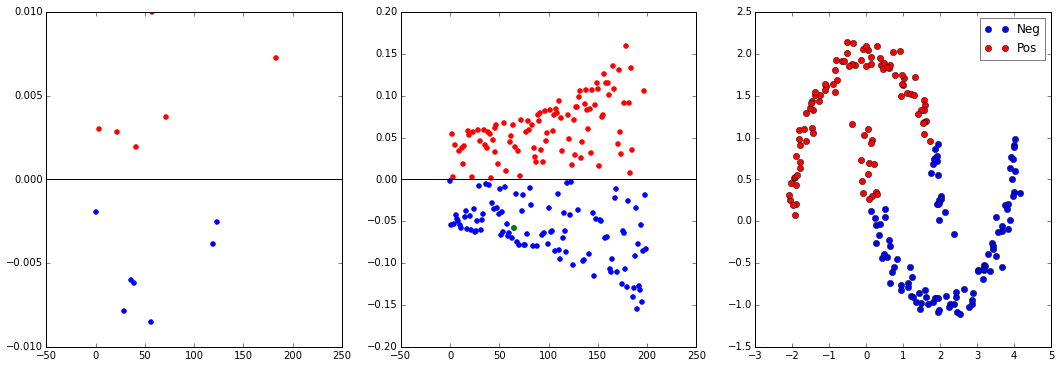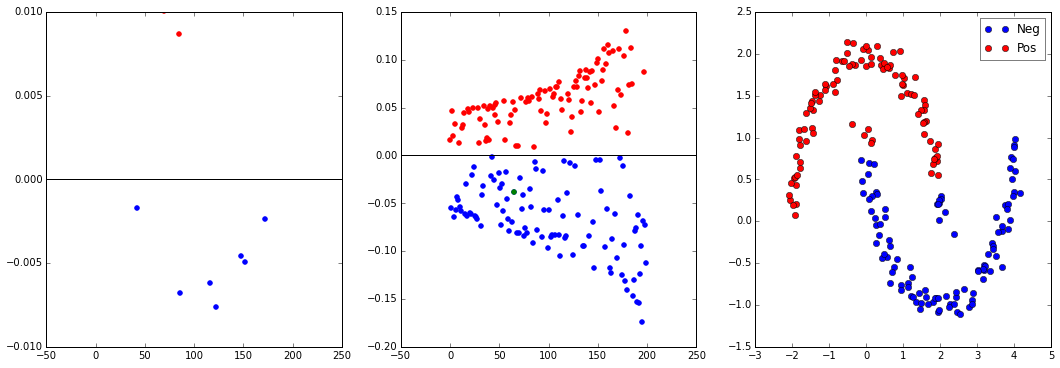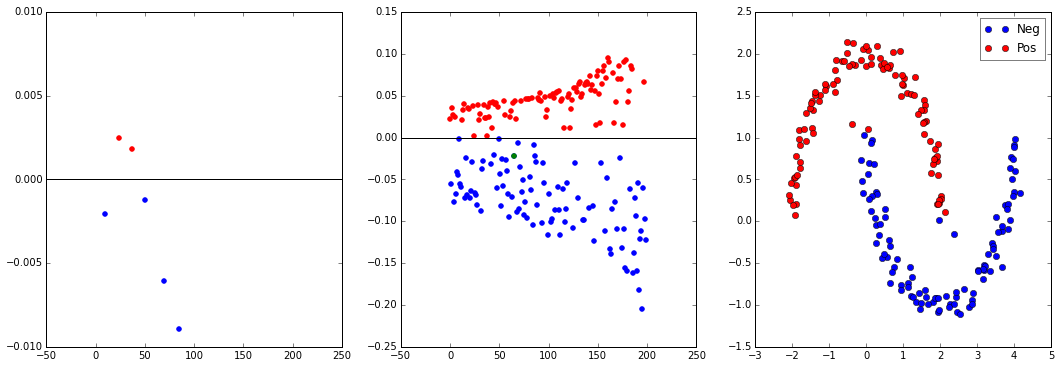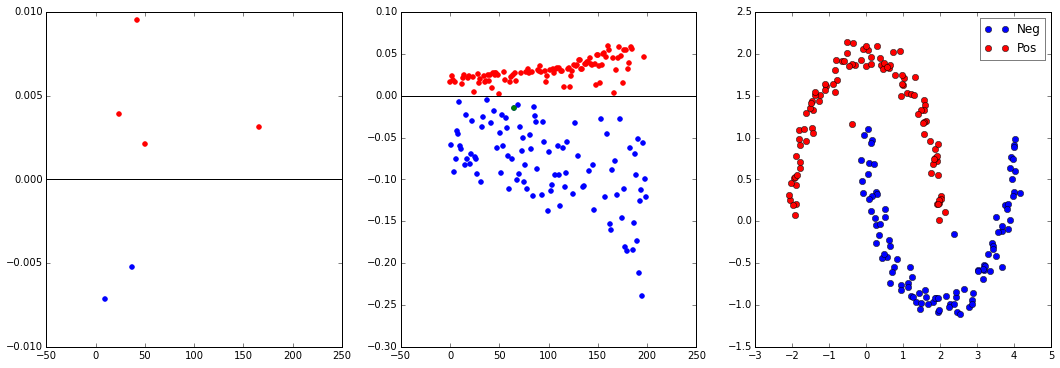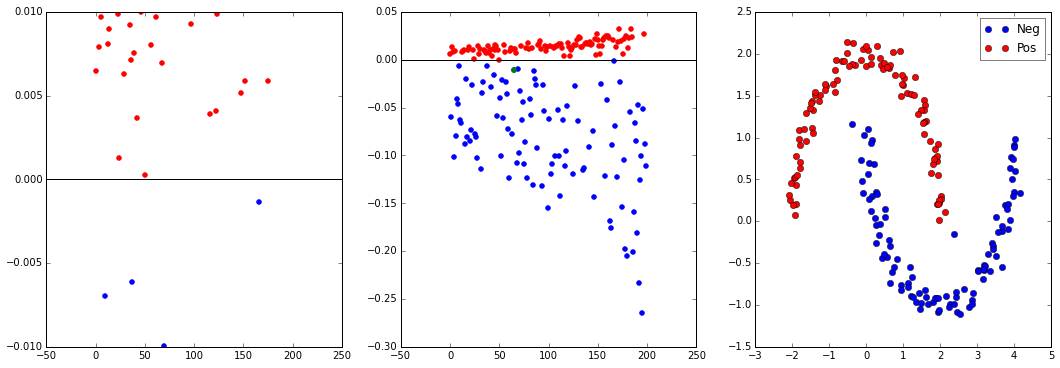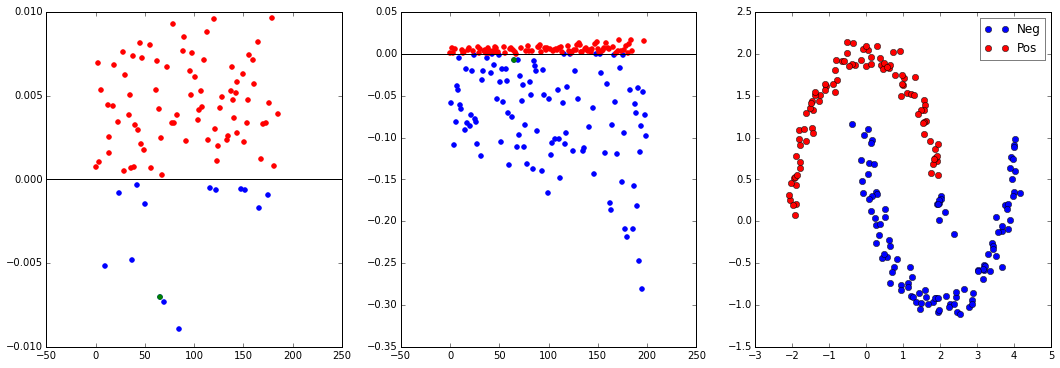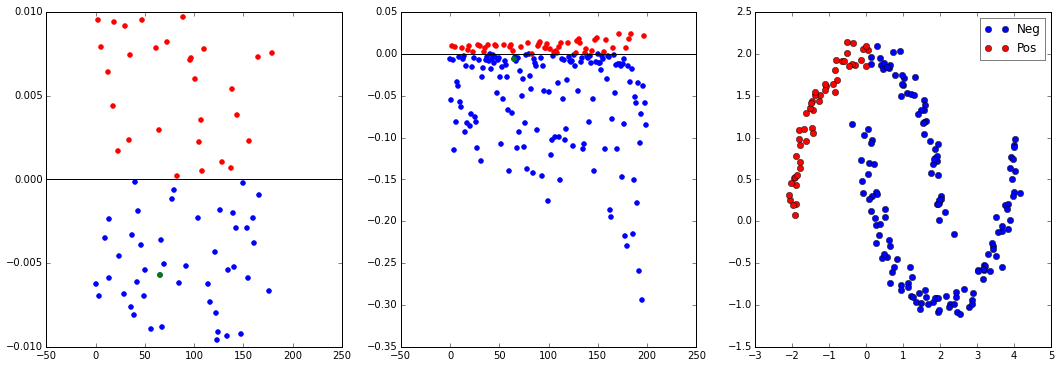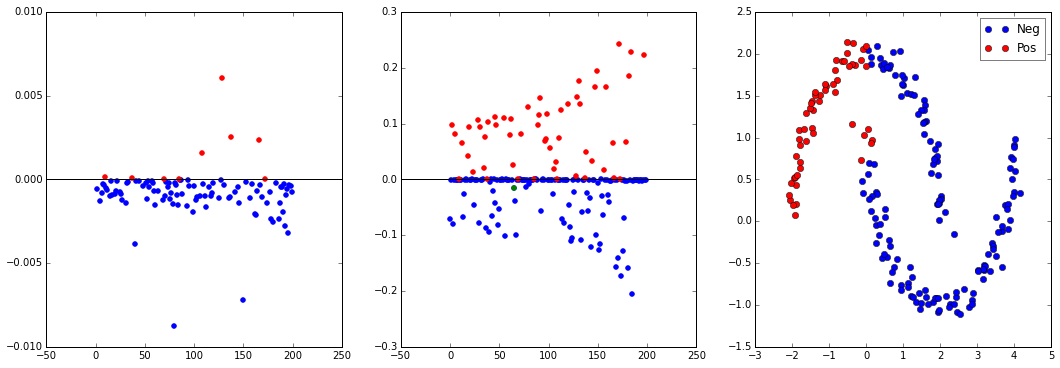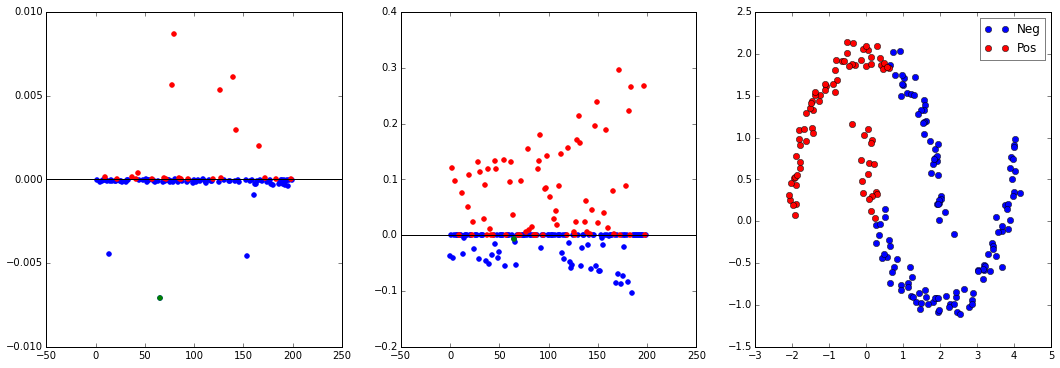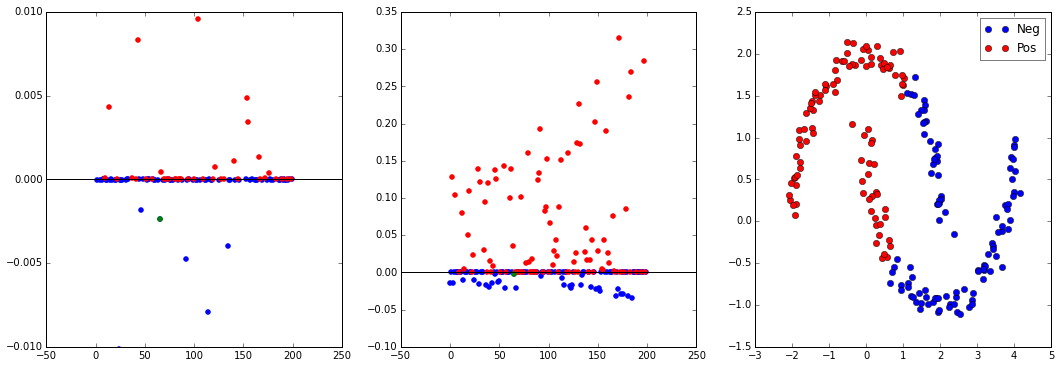# Project 3¶

## B-IT Pattern Recognition¶

Presented on 4-Feb-2016 by:

• Abdullah Abdullah

• Can Güney Aksakallı

• Kang Cifong

• Umut Hatipoğlu

### The Data¶

In :
mydata = demo_1_data()

200 samples of 2 dimensional data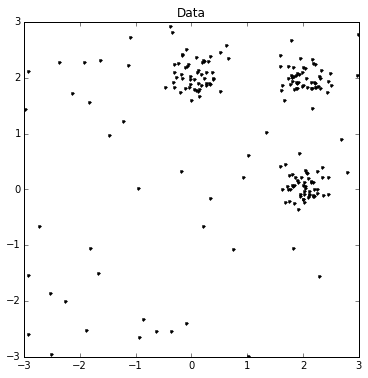### Lloyd's Algorithm¶

• Very Sensitive to initialization values

• Converges, but no guarantees (esp in case of bad initializations)

• No Guarantee about the results either

• Really Fast (if no catastrophy)

In :
demo_1_lloyd(mydata, 3)

Converged after 4 iterations
Converged after 9 iterations
Converged after 3 iterations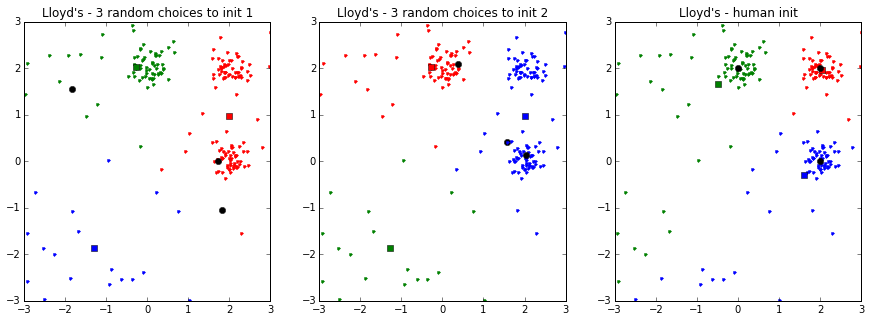### Different Similarity Measures¶

• The data does seem to have Gaussian Blobs

• The problem with the data is different
• Different similarity metric will probably not give different results

• Except in case of relatively bad similarity metrics
In :
demo_1_lloyd2(mydata, 3, ['euclidean', 'cityblock', 'mahalanobis'], 800)
demo_1_lloyd2(mydata, 3, ['euclidean', 'cityblock', 'mahalanobis'], 10)

Converged after 5 iterations
Converged after 6 iterations
Converged after 5 iterations
Converged after 5 iterations
Converged after 4 iterations
Converged after 5 iterations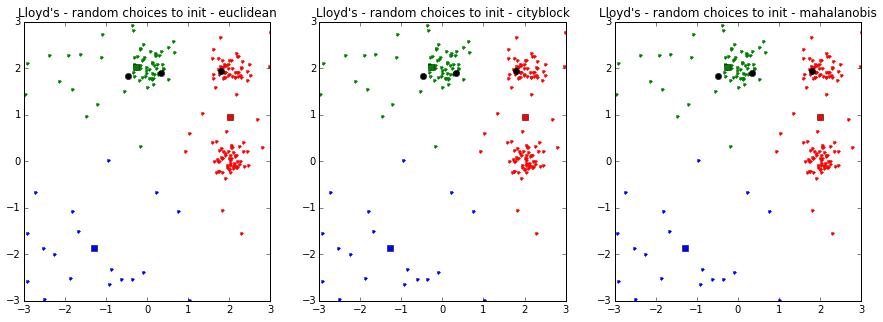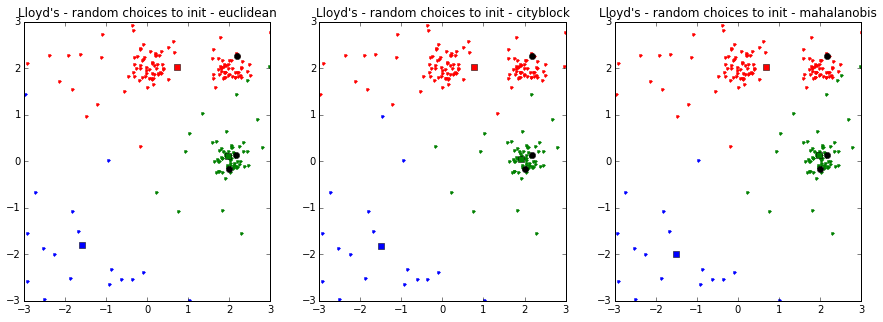### Hartigan's Algorithm¶

• Converges quickly

• Relatively Robust

• Still sensitive to initialization of classes

In :
demo_1_hart(mydata, 3, [12, 42, 999])  # These took some time to choose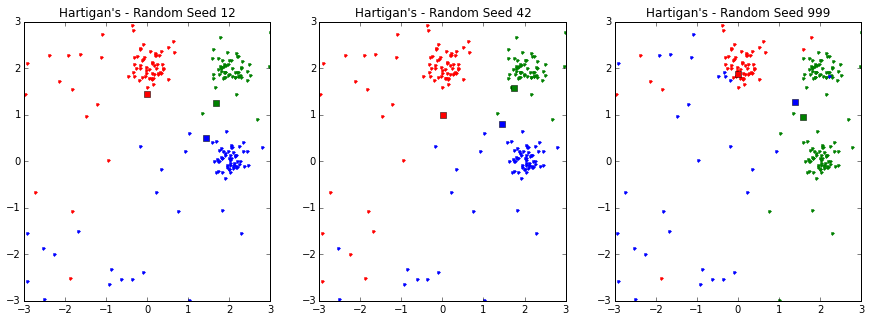#### Smarter Way?¶

• We couldn't figure out any smarter way, rather than :

• only recalculate objective function for the current class

• Not reliable, esp when the data is disproportionate among classes
• calculate the change in objective function incrementally

• Halved the number of data points for which the distance is calculated, compared to Naive

• Does not fully utilize the potential, eg vectorization

m[ki] = (n[ki] / (n[ki] - 1)) * (m[ki] - x / n[ki])

normxkk = norm(x - m, axis=1)
eki = np.sum(norm(Xki - m[ki])) - normxkk[ki]
ediffki = eki - e[ki]

ediff = ediffki + normxkk

kw = np.argmin(ediff)


### MacQueen's Algorithm¶

• Convenient for streams

• Sensitive to order of data

• Essentially, still sensitive to initialization
In :
demo_1_macqueen(mydata, 3, [12, 556])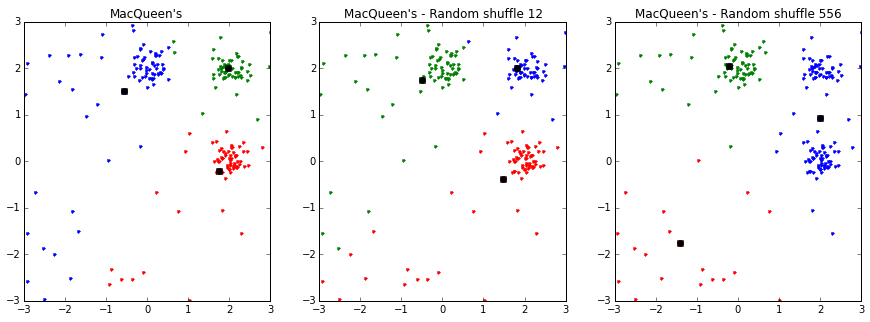### Run times!¶

In :
np.random.seed(9000)
init_c = np.copy(mydata[np.random.choice(np.arange(mydata.shape), size=3)])
demo_1_t()

Mac OSX - 10.11.3
2,9 GHz Intel Core i7 (On Battery)
Python 3.4

Lloyd - Naive
10 loops, best of 3: 57.9 ms per loop

Lloyd - 2
1000 loops, best of 3: 972 µs per loop

Lloyd - sklearn.cluster.KMeans
1000 loops, best of 3: 1.82 ms per loop

?? - scipy.cluster.vq.kmeans
1000 loops, best of 3: 683 µs per loop

?? - scipy.cluster.vq.kmeans2
1000 loops, best of 3: 796 µs per loop

Hartigan - Naive
1 loops, best of 3: 332 ms per loop

Hartigan - 2
10 loops, best of 3: 52 ms per loop

MacQueen - Naive
100 loops, best of 3: 17.2 ms per loop

MacQueen - 2 (numpy-ed)
100 loops, best of 3: 8.88 ms per loop

/Users/myrmidon/.conda/envs/pattrex/lib/python3.4/site-packages/sklearn/cluster/k_means_.py:794: RuntimeWarning: Explicit initial center position passed: performing only one init in k-means instead of n_init=10
n_jobs=self.n_jobs)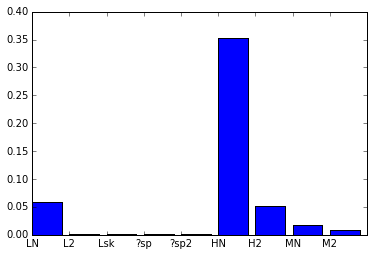### Syllabus¶

• Apply K-means

• Apply Spectral Clustering

• Apply Spectral Clustering using Andrew Ng's Alorithm

In :
my_data = demo_2_1()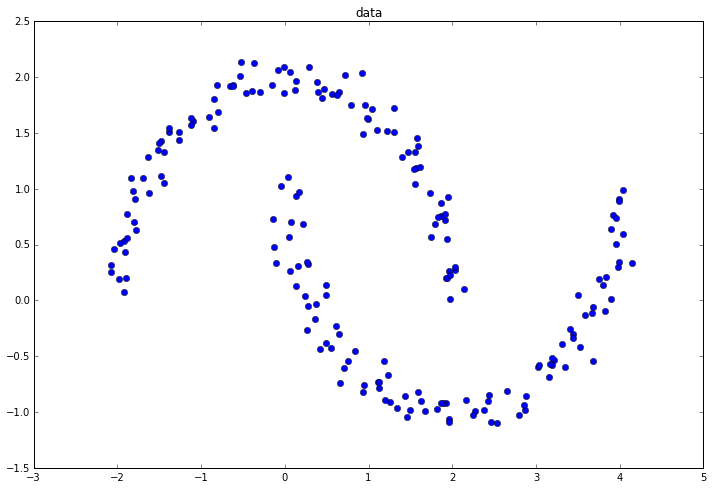### Apply K-means on Data¶

In :
demo_2_2(my_data)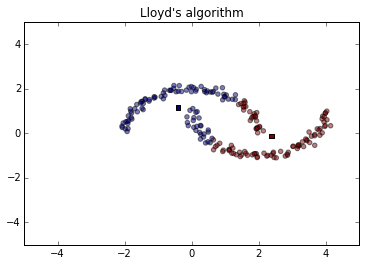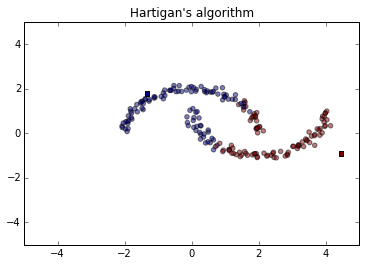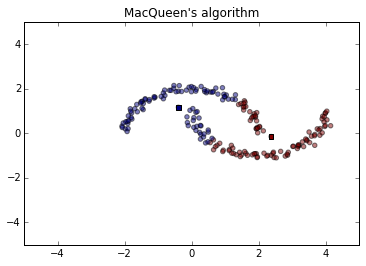## Apply Spectral Clustering on Data¶

• Get a good result at beta = 11
• By observation, we see that some edges points would be mis-judged as beta grows from 1 to 15
• The Upper half contains 100 points, and so is the lower half.

### Play around the number of halfs¶

• See how the number of halfs changes
In :
demo_2_4(my_data)

1 103
2 103
3 101
4 100
Got 4  with 100 each
5 100
Got 5  with 100 each
6 101
7 101
8 109
9 124
10 106
11 103
12 104
13 114
14 118
15 120
16 122
17 122
18 122
19 107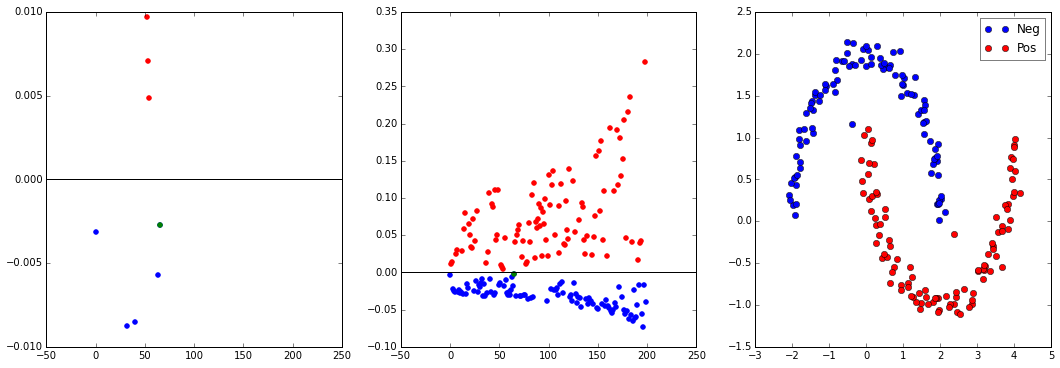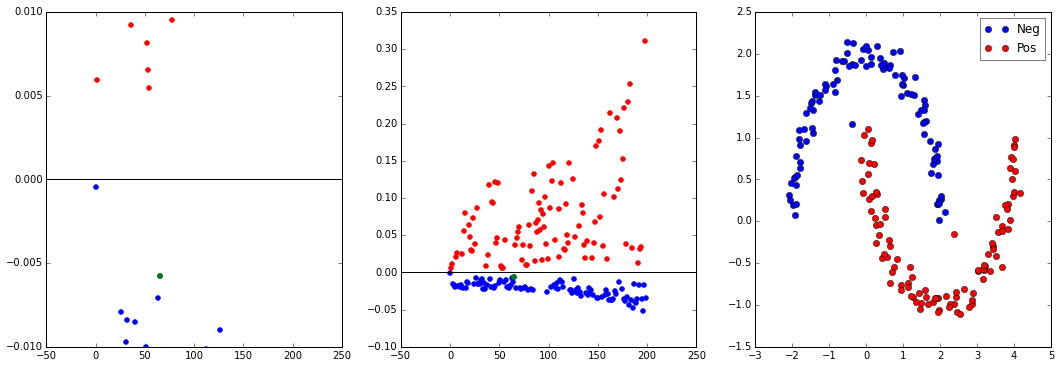## Exam the Laplacian Matrix¶

• S = exp(-beta* |x_i-x_j|^2) which is indepandent on the data order
• D = Sum(j to n)(Sij) if i=j which is depandent on the data order
• L = D - S

### Shuffle the data order to see the result¶

• we would have a differnet beta or even unable to get one sometime. Sometimes we got a lot
• But we see that the upper half gathered close to y=0 line, while the lower half spread around.
In :
demo_2_5(my_data)

Got 3
Got 4